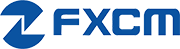AUD/JPYAustralian Dollar / Japanese Yen
Markets
Closed
Prices byPrice
1DChange
1DChange

## AUD/JPY Pivot Points

Pivot Points help identify potential support and resistance levels.

= Current Price

### Understanding Pivot Points

Pivot points help to identify potential support and resistance levels, which are areas where the price can potentially change direction.

There are different types of pivot points. Each with its own formula on how to calculate support and resistance levels. They are popular tools for short-term traders looking to take advantage of intraday price movements.

To learn more about how pivot points work, check out our lesson, "How to use Pivot Points".

#### Pivot Point Meters & Charts

= Current Price#### How Pivot Points are Calculated

##### Floor

The floor pivot points are the most basic and popular type of pivots. The pivot point is interpreted as the primary support/resistance level - the point at which the main trend is determined. First-third level resistance and support points serve as additional indicators of possible trend reversal or continuation.

```Pivot (P) = (H + L + C) / 3
Resistance (R1) = (2 x P) - L
R2 = P + H - L
R3 = H + 2 x (P - L)
Support (S1) = (2 x P) - H
S2 = P - H + L
S3 = L - 2 x (H - P)```
##### Woodie

Woodie's pivot points are similar to floor pivot points, the difference being is that more weight is given to the Close price of the previous period.

```Pivot (P) = (H + L + 2 x C) / 4
Resistance (R1) = (2 x P) - L
R2 = P + H - L
Support (S1) = (2 x P) - H
S2 = P - H + L```
##### Camarilla

Camarilla pivot points are a set of eight very probable levels which resemble support and resistance values for a current trend. The most important is that these pivot points work for all traders and help in setting the right stop-loss and profit-target orders.

```R4 = (H - L) x 1.1 / 2 + C
R3 = (H - L) x 1.1 / 4 + C
R2 = (H - L) x 1.1 / 6 + C
R1 = (H - L) x 1.1 / 12 + C
S1 = C - (H - L) x 1.1 / 12
S2 = C - (H - L) x 1.1 / 6
S3 = C - (H - L) x 1.1 / 4
S4 = C - (H - L) x 1.1 / 2```
##### Tom DeMark's

Another popular method of calculating the pivots to forecast the future of the trend is Tom DeMark's pivot points, which are not pivot points exactly, but are the predicted lows and highs of the period.

```If Close < Open: X = H + (2 x L) + C
If Close > Open: X = (2 x H) + L + C
If Close = Open: X = H + L + (2 x C)
R1 = (X / 2) - L
S1 = (X / 2) - H```
##### Fibonacci

Fibonacci pivot point levels are determined by first calculating the floor pivot points. Next, multiply the previous day's range with its corresponding Fibonacci level. Most traders use the 38.2%, 61.8% and 100% retracements in their calculations. Finally, add or subtract the figures you get to the pivot point and voila, you've got your Fibonacci pivot point levels!

```R3 = PP + ((High - Low) x 1.000)
R2 = PP + ((High - Low) x 0.618)
R1 = PP + ((High - Low) x 0.382)
PP = (H + L + C) / 3
S1 = PP - ((High - Low) x 0.382)
S2 = PP - ((High - Low) x 0.618)
S3 = PP - ((High - Low) x 1.000)```